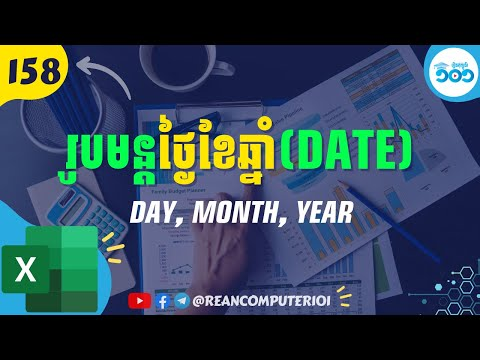## How to use DAY MONTH YEAR and DATE in Excel with Example Download

If you want to get separate day, month and year from the date, you can use DAY, MONTH and YEAR formulas in Excel to retrieve the information. If you have input separate cells of day, month, and year and want to put together and can use as DATE format, then DATE formula is used.### DAY formula in Excel

DAY returns the numbers of the month as integer ( value from 1 to 31).
FORMULA:
=DAY(serial_number)

◾ Serial_number: Required. Basically is Date.

### MONTH formula in Excel

MONTH returns the numbers of two month as integer ( value from 1 to 12).
FORMULA:
=MONTH(serial_number)

◾ Serial_number: Required. Basically is Date.

### YEAR formula in Excel

YEAR returns the numbers as year from the input date (value as an integer from 1900-9999).
FORMULA:
=YEAR(serial_number)

◾ Serial_number: Required. Basically is Date.

### DATE formula in Excel

DATE returns the serial number that represent as date. Basically DATE fomula combine day, month and year together as date format.
FORMULA:
=DATE(year,month,month)

◾ year Required. The value of the year.
◾ month Required. The value of the months.
◾ day Required. The value of the days.

Watch How to use DAY MONTH YEAR and DATE in Excel speak Khmer